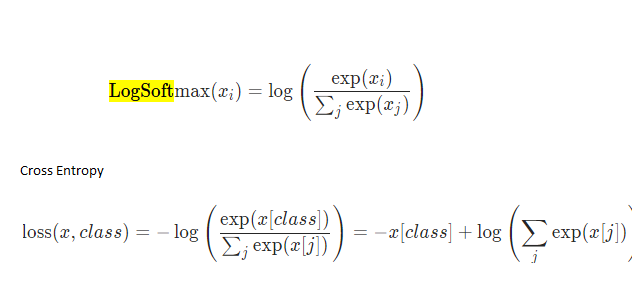# Sigmoid and BCELoss

Got this from the documentation

m = nn.Sigmoid()
loss = nn.BCELoss()

#input is of size N x C = 1 x 3

print(input)

#each element in target has to have 0 <= value < C
target = torch.empty(3).random_(2)

print(m(input))
print(target)

output = loss(m(input), target)

print(output)

OUTPUT

tensor([0., 1., 0.])

Questions
This is the values after sigmoid which is btw 0,1
[0.2923, 0.6749, 0.3580] <-- is this 3 y-predictions ?

this is y-actual
tensor([0., 1., 0.])

In this case since the 2nd element will round to 1 we predict correctly for all the 3 elements

How does loss compare both and obtain 0.394?

Thanks

Hi Snip!

Yes. But these should be understood as probabilistic predictions.
That is, you are predicting a 29% chance of being in class “1” (and
hence a 71% chance of being in class “0”), a 67% chance of being
in class “1”, and a 36% chance of being in class “1”, respectively.

Yes.*

Yes, but you have note that you are converting from probabilistic to
deterministic yes-no predictions. Rounding (that is, testing that `p > 1/2`)
yields `[0, 1, 0]`. This means class “0”, class “1”, class “0”, with zero
predicted probability of being in the other class. This is the right thing
to do when you want to measure the accuracy of your classifier, but
doesn’t really work as a loss function used for training.

In the code you posted `BCELoss` is comparing what you call “y-actual”
with the probabilistic prediction, and is not rounding anything to obtain
yes-no predictions.

If your probabilistic prediction were `[0.0, 1.0, 0.0]`, then it would be
a perfect prediction (You would be predicting y-actual with 100%
certainty.), and the loss would be 0.0.

But, for example, the first entry of your prediction is `0.29`. So you
are predicting the correct y-actual – class “0” – with 71% certainty.
That’s pretty good, but not “perfect.” And you are incorrectly predicting
class “1” – not the y-actual – with a 29% chance, still pretty good, but
partially wrong.

So your loss is greater than 0, reflecting your imperfect (but pretty
good) prediction.

*) When training binary classifiers with `BCELoss` our targets / labels
are usually `0.0` and `1.0` and can be interpreted as class “0” and
class “1” class labels. But they can also be understood as the
probability of being in class “1”, and are only required by `BCELoss`
to be in the range `0.0 <= p <= 1.0`. Technically, `BCELoss` is
calculating the cross-entropy between two probability distributions,
the predicted probability, and the target (y-actual) probability. The
details can be found in the BCELoss documentation.

(As a side note, in practice you are better off leaving out the `Sigmoid`,
and using `BCEWithLogitsLoss`, that has, in effect, `Sigmoid` built in.)

Best.

K. Frank

Hi @KFrank

I think I got it already

But I have some confusion for crossentropy.I kinda understand regarding the dim=1 which came from the screenshot below.We need to sum up the denominator for both but why we do not require dim for CrossEntropyLossIs my understanding correct when I try so simulate a bad prediction and a good one?
For the target(y_actual),we need to do one hot encoding too.

Also, how can we simulate the NLL?

we need to exp and then multiply by -1

Sidetrack, in DL if we’re saying logits does it mean raw form as in our input in the codes?
after we apply sigmoid or softmax, it will be probability?

Thanks.